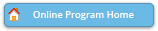#### Abstract Details

 Activity Number: 311 Type: Contributed Date/Time: Tuesday, August 2, 2016 : 8:30 AM to 10:20 AM Sponsor: Biometrics Section Abstract #319487 Title: Joint Modeling for Logistic Regression Models with Generalized Method of Moments Estimators Author(s): Katherine Cai* and Jeffrey Wilson Companies: Arizona State University and Arizona State University Keywords: joint modeling of the mean and variance ; double generalized linear models ; correlated data ; generalized method of moments ; simulation ; logistic regression Abstract: Generalized linear models often have a specified relationship between the mean and the variance. While it is common for the dispersion to be a function of the mean of a random variable, the data may not have the exact mean-variance relationship as assumed by the probability distribution. Smyth (1989) demonstrated that generalized linear models can be conveniently expressed as separate functions of the mean and dispersion. This technique, joint modeling of the mean and dispersion, uses the deviance from the mean model as the dependent variable for the dispersion model. Joint modeling accounts for additional variation that is not explained by the mean model and is of particular interest when overdispersion is present. We examine joint modeling in the context of logistic regression models and investigate generalized method of moments (GMM) as an alternative estimation technique. We present a comparison of both the likelihood and GMM methods using simulations and a numerical example.

Authors who are presenting talks have a * after their name.